### Light Reflection and Refraction - Test Papers

CBSE Test Paper-01

Chapter 10 Light Reflection and Refraction

1. A student performs an experiment on finding the focal length of a convex lens by keeping a lighted candle on one end of laboratory table, a screen on its other end and the lens between them as shown in the figure. The positions of the three are adjusted to get a sharp image of the candle flame on the screen.If now the candle flame were to the replaced by a distant lamp on a far away electric pole, the student would be able to get a sharp image of this distant lamp on the screen by moving (1)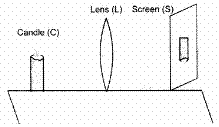1. the screen in the direction of the lens or the lens away from the screen
2. neither the screen nor the lens
3. the screen in the direction of the lens or the lens in the direction of the screen
4. the screen away fro the lens or the lens in the direction of the screen
2. Four students set up the glass slab experiment as shown below: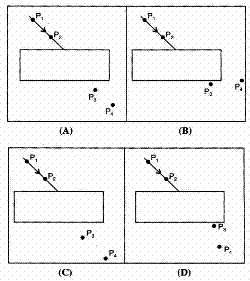The correct fixing of the pins Pand P, for 'defining the incident ray', and of pins P3 and P4, for finding the emergent ray', has been done by student: (1)

1. A
2. C
3. B
4. D
3. When a lemon kept in water in a bowl is viewed from outside, it appears ___________ than its actual size. (1)

1. None of these
2. Smaller
3. Larger
4. Same
4. As incident angle is increased for a given pair of medium, the refraction angle will (1)

1. decrease
2. always remains same
3. may increase or decrease
4. increase
5. An object move a distance 'f' between 2f and f of a concave mirror. The image would have travelled a distance of (1)

1. $\frac{f}{2}$
2. 2f
3. f
6. What is a ray of light? (1)

7. What is the name given to the centre of the mirror ? (1)

8. What is an optically denser medium? (1)

9. Define the term angle of incidence. (1)

10. State two factors which determine lateral displacement of a ray of light passing through a rectangular glass slab. (3)

11. Draw ray diagram showing the image formation by a convex lens when an object is placed at twice the focal length of the lens. (3)

12. A concave lens has a focal length of 10 cm. An object 2.5 cm high is placed 30 cm from the lens. Determine the position and size of the image. (3)

13. Two friends Kapil and Rohit were studying in the same class. One day Rohit observed that Kapil was having pain in gums during lunch time. Rohit told Kapil that his father was dentist and asked him to visit his father's clinic. Rohit's father examined Kapil with the help of a mirror and advised him not to eat too many chocolates and soft drinks. Kapil follow ed the advise of the doctor and soon he got recovered. After that he starts taking care of his mouth, as he washes his mouth properly after every meal and also starts taking a calcium rich diet. Read the given passage and answer the following questions: (3)

1. Identify the mirror used by the dentist.
2. Name the phenomenon of light by which doctor is able to exam ine Kapil.
3. What values are shown by doctor, his son and Kapil?
14. Define the principal focus of concave mirror. (5)

15. How are the images formed when an object is moved from infinity to the convex lens? (5)

CBSE Test Paper-01
Chapter 10 Light Reflection and Refraction

1. the screen in the direction of the lens or the lens in the direction of the screen
Explanation: The candle is closer to the lens when compared to the distant lamp. Hence, the image of the candle will be farther away from the lens when compared to the image of the distant lamp. As the image will be more close to focus as the distance of the object is larger.
1. C
Explanation: Emergent light is parallel to the incident light and laterally displaced.
1. Larger
Explanation: When a lemon is kept in water in a bowl, and is viewed from the outside, it appears larger than its actual size due to refraction. When reflected rays of light travel from the lemon (which is in water) towards the eye (which is in air), the rays travel from a denser medium to a rarer medium and they bend away from the normal to the water surface.
1. increase
Explanation: Since $n=\frac{\mathrm{sin}i}{\mathrm{sin}r}$= constant, with increase in i, r will increase.

1. Explanation: Image of an object at 2f will be at 2f, while it will be at ∞, if object is at f.
1. The path along which light travels is called a ray of light.

2. The centre of reflecting surface of a spherical mirror is known as Pole.

3. A medium in which light travels comparatively slower than the other medium is called an optically denser medium.

4. The angle between an incident ray and the normal at the point of incidence is called angle of incidence.

5. 2 Factors which determine lateral displacement are as given below:

1. Lateral displacement is directly proportional to the thickness of glass slab.
2. Lateral displacement is directly proportional to the angle of incidence.
6.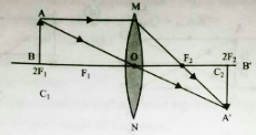7. Since the lens is concave, hence f is negative
Given: u = - 30 cm; f= - 10 cm; h = 2.5 cm; v = ?; h' = ?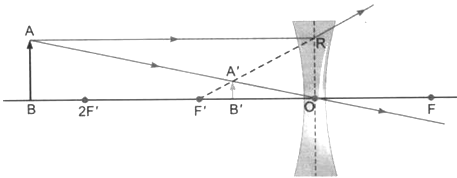The lens formula for concave lens is $\frac{1}{v}-\frac{1}{u}=\frac{1}{f}$
$\frac{1}{v}-\frac{1}{-30}=\frac{1}{-10}$
$\frac{1}{v}=\frac{1}{10}-\frac{1}{30}$
$\frac{1}{v}=\frac{-3-1}{30}$
v = -7.5 cm
The image is at a distance of 7.5 cm from lens (in front of lens).
The magnification m = $\frac{v}{u}=\frac{-7.5}{30}$
$\frac{1}{4}$
= +0.25
The positive sign with the magnification indicates that the image formed erect.
The size of the image is determined by h'.
$\frac{{h}^{\prime }}{h}$ = m
h' = $h×m$
$2.5×0.25$
= 0.625 m
Thus the image formed is virtual and erect. It is at a distance of 7.5 cm from lens and its size is 0.625 cm.

1. The mirror used by the dentist is concave mirror.
2. The phenomenon of light by which doctor is able to examine Kapil is reflection of light.
3. The doctor gave the correct advise to Kapil on how to keep his mouth clean and gums healthy. The doctor’s son and doctor was helping in nature. Kapil followed the advise given by the doctor. So, he is an obedient boy.
8. Light rays that are parallel to the principal axis of a concave mirror converge at a specific point on its principal axis after reflecting from the mirror. This point is known as the principal focus of the concave mirror.
Principal focus (p.f.) is a point on principal axis of a concave mirror where the rays parallel to principal axis meet after reflection from the mirror.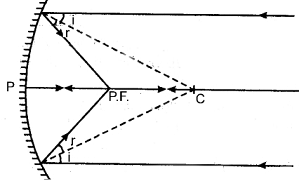Additional reading. For convex mirror, principal focus is a point on principal axis of a convex mirror where rays parallel to principal axis appear to diverge from after reflection from the mirror.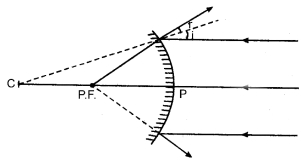9. Object at Infinity. When object is at infinity, a real image is formed at F on the other side of the lens
​​​​​​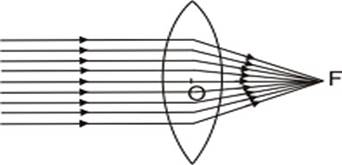Object at infinity. Image at F on the other side of lens.
However if the rays are parallel to themselves but not parallel to principal axis, then these rays after refraction will form image at focus F' and not at principal focus F.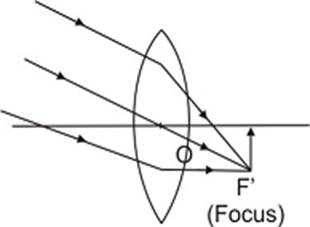Object at infinity, rays parallel to themselves but not parallel to principal axis. Image is formed at F', the focus on the other side of lens. Object beyond 2F. When the object is beyond 2F, a real, inverted, diminished image is formed between F and 2F.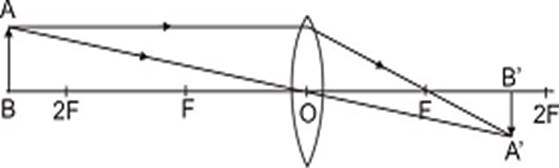Object beyond 2F, real, inverted, diminished image between F and 2F. Object at 2F. When the object is at 2F, a real, inverted image of the same size is formed on the other side of the lens at 2F as given in Fig.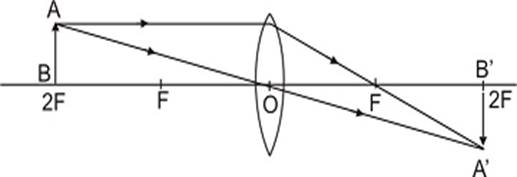Object at 2F, image at 2F on the other side of the lens. Image is of size same as that of the object. When the object is between F and 2F, its real, inverted, magnified image is formed on the other side of the convex lens as shown in fig.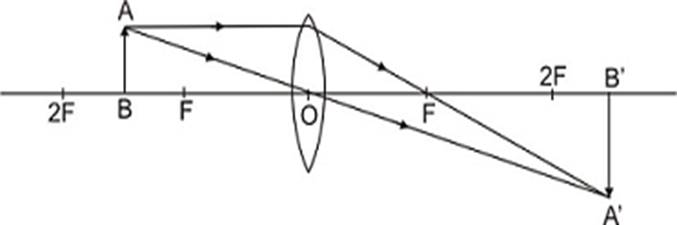Object between F and 2F real, inverted, magnified image is formed beyond 2F on the other side of lens.
Object at F. When object is placed principal focus, a real, inverted, very highly magnified image is formed at infinity.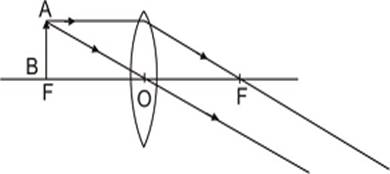Object at F, a very highly magnified, real, inverted image is formed at infinity.
Object between F and C. When an object is placed between principal focus and optical centre of the lens, virtual, erect, magnified image is formed on the same side of the lens.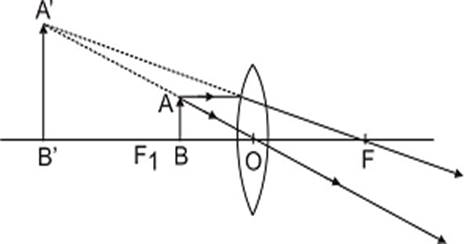Object between F and C; a virtual, erect, magnified image is formed on the same side.